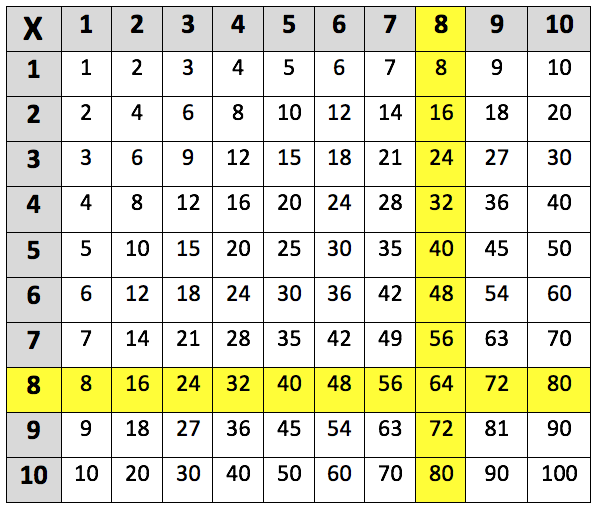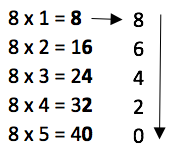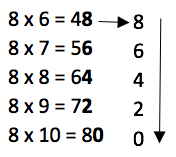Learning the 8 times table. The 8 times table up to 8 x 10 is highlighted in the multiplication grid below:This is the 8 times table up to 8 x 12:
8 x 1 = 8
8 x 2 = 16
8 x 3 = 24
8 x 4 = 32
8 x 5 = 40
8 x 6 = 48
8 x 7 = 56
8 x 8 = 64
8 x 9 = 72
8 x 10 = 80
8 x 11 = 88
8 x 12 = 96

## When do children learn the 8 times table?

In Year 2, children are taught the concept of multiplication and start by learning the 2, 5 and 10 times tables.

In Year 3, teachers move on to the 3, 4 and 8 times tables. Children should also be able to use practical and written methods of multiplying and dividing two-digit numbers. For example:

12 x 8 = 96

## Are there any tricks to make the 8 times table easier to learn?

There are a few helpful tips to help children learn the 8 times table, such as the fact that each multiple of 8 is an even number. There is also a pattern noticeable in the digits of multiples of 8, they go down in 2s. For example:Therefore, it could be easier for children to learn the 8 times table by breaking it down into two parts, like above.

## How to help children with the 8 times table?

Sometimes the process of paired reading can help a child learn their times tables, as repeating tables together out loud will give children confidence in reading multiplication grids. This method can work because children often learn through repetition and it will also help them with fluency.

If children are struggling with a particular times table, it may be useful to remind them that they can reverse the order of a multiplication. For example:

8 x 5 is the same as 5 x 8 (both = 40)

This method will benefit children who are more confident with the other times tables, such as 5.

## Why is learning the times tables so important?

It is very important that children are confident with their times tables in Year 5 and 6. This is because their knowledge of multiplication will help them understand more complex mathematical concepts, such as problem solving, percentages and fractions. If children do not know their times tables well enough, they may start to struggle when teachers introduce tricky concepts such as algebra equations in Year 6.

Knowing their times tables will not just help children at primary school, but will also make learning in secondary school easier. Multiplication skills can be useful for subjects other than maths, such as the 3 science subjects (physics, biology, chemistry) and in IT.

## How does Learning Street help children with the 8 times table?

Repetition is key to learning your 8 times table, and in the Learning Street 8 year old courses, there is constant focus on always practicing times tables as this is the best way to improve. This is through times tables tests (to improve accuracy), tables races (to improve time), mental mathematics, maths problem solving and other fun activities such as mystery pictures.

Without a good knowledge of times tables, it can lead to problems in SATs, GCSEs and A Levels.

## Our Courses

Click through to review the courses we have available

##### Get Started

Learning Street structured courses make home study easier and more successful. There's no need to wonder which books to buy or what to do next. Everything is planned for you.

• Proven to deliver excellent results
• Used by families, private tutors and schools
• Fully planned and structured